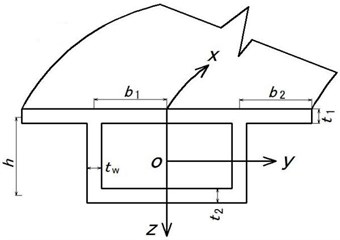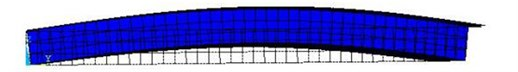Published: 30 June 2017

# Free vibration analysis of thin-walled curved box girder considering shear lag deformation

Heng Cai1
Hailin Lu2
Hongyin Yang3
1, 2, 3School of Resource and Civil Engineering, Wuhan Institute of Technology, Wuhan, China
Corresponding Author:
Hailin Lu
Views 57

#### Abstract

In order to investigate dynamic shear lag effect, based on energy variation method and Hamilton principle, vibration governed differential equations of curved box girder are deduced by assuming different shear lag warping displacement modes, the explicit solution to bending frequency of curved box girder is worked out with Galerkin method. The results of a numerical example show that shear lag warping displacement functions have a limit influence on vibration frequency; second degree parabola or catenary are the appropriate shear lag warping displacement function of box girder; Compared the theoretical calculating results with numerical results of ANSYS, the error between them is only 0.57 %, they agree very well with each other, which demonstrates the correctness and reliability of theoretical deduction.

## 1. Introduction

Thin-walled box girder with wide flange is widely applied in the engineering construction for its merits such as great stiffness, light weight and so on. While shear lag effect  is a prominent problem in box girder, it means that normal stress of flange is non-uniform distributed along width direction because of flange shear deformation. This phenomenon will affect the safety and durability of box girder bridges . Energy variation method, based on principle of minimum potential energy, can not only acquire closed-form solution [3, 4] but also get the real peak stress at the ribs, therefore it is widely applied in calculating shear lag.

Bridges will bear vehicle load, wind load, and sometimes seismic action when they are in service, under the impact of vehicle load and vertical seismic excitation, box girder considered curvature will create strong coupling effect of bending, torsion and shear lag effect, which will make it difficult to calculate its internal force and deformation under dynamic loads, while vibration frequency is an important parameter to reflect the dynamic characteristics of bridges especially when General code for design of highway Bridges and culverts  is used to calculate impact factor. Shear lag warping displacement mode is the premise and basis of energy variation method, because it can influence solution accuracy. In this paper, shear lag warping displacement modes are respectively assumed as parabola series [6-8], cosine function, exponential function and catenary, governed differential equations of curved box girder are deduced by energy variation method and Hamilton principle and the explicit solution is also acquired, meanwhile, shear lag warping displacement modes with high precision are studied.

## 2. System potential energy

In order to simplify mechanic model, assumptions are as following:

(1) The damp is not considered and the material is linear elastic.

(2) The flanges don’t conform to plane cross-section assumption while the webs do.

(3) Shear lag effect is only considered in vertical bending deformation.

(4) Transverse deformation and shear deformation in out-plane are neglected, they mean ${\epsilon }_{y}={\epsilon }_{z}=0$, ${\gamma }_{yz}={\gamma }_{xz}=0$.

The cross section of curved box girder is shown as Fig. 1.

Fig. 1Cross section type of curved box girderAccording to the assumptions above, the longitudinal shear lag warping displacement is shown as Eq. (1):

1
$u\left(x,y,t\right)=\zeta \left(y\right)u\left(x,t\right),$

where $u\left(x,t\right)$ is maximum difference shear angle of flange and $\zeta \left(y\right)$ is warping function, they are assumed to be as following:

(1) Parabola series:

2
$\zeta \left(y\right)=1-{\left(\frac{y}{{b}_{i}^{}}\right)}^{n},\left(n=2,3,4,5\right).$

(2) Cosine function:

3
$\zeta \left(y\right)=\mathrm{c}\mathrm{o}\mathrm{s}\left(\frac{\pi }{2}\frac{y}{{b}_{i}}\right).$

(3) Exponential function:

4
$\zeta \left(y\right)=\frac{e-{e}^{y/{b}_{i}}}{e-1}.$

(4) Catenary:

5
$\zeta \left(y\right)=2-\mathrm{c}\mathrm{o}\mathrm{s}\mathrm{h}\left(\eta y\right),\mathrm{}\mathrm{}\mathrm{}\eta =\frac{\mathrm{l}\mathrm{n}\left(2+\sqrt{3}\right)}{{b}_{i}},$

where ${b}_{i}\left(i=1,2\right)$ are the half flange width and cantilever slab width respectively.

The total potential energy of curved box girder is shown as Eq. (6):

$U={U}_{w}+{U}_{f}+{U}_{t}+{U}_{r}=\frac{EI}{2}\underset{0}{\overset{l}{\int }}\left({w}^{\text{'}\text{'}}-\frac{\varphi }{r}{\right)}^{2}dx+\frac{G{I}_{t}}{2}\underset{0}{\overset{l}{\int }}\left({\varphi }^{\text{'}}+\frac{{w}^{\text{'}}}{r}{\right)}^{2}dx\begin{array}{l}+\frac{E{I}_{w}}{2}\underset{0}{\overset{l}{\int }}{\left({\varphi }^{\text{'}\text{'}}+\frac{{w}^{\text{'}\text{'}}}{r}\right)}^{2}dx+\frac{G\overline{{I}_{s}}}{2}\\ \end{array}$
6
$\underset{0}{\overset{l}{\int }}\frac{4{u}^{2}}{3{b}^{2}}dx+\frac{E{I}_{s}}{2}\underset{0}{\overset{l}{\int }}{\left({w}^{\text{'}\text{'}}-\frac{\varphi }{r}\right)}^{2}+\frac{8}{15}{u}^{{\text{'}}^{2}}+\frac{3}{2}{u}^{\text{'}}\left({w}^{\text{'}\text{'}}-\frac{3\varphi }{2r}\right)dx,$

where ${U}_{w}$, ${U}_{f}$ are web and flange energy, and ${U}_{t}$, ${U}_{r}$ are free torsion and restricted torsion potential energy respectively, $w$, $\varphi$ are vertical deflection and torsion angle, $r$ is curvature radius, ${h}_{i}\left(i=1,2\right)$ are the distances from cross section center to the upper and bottom flange. Besides, $E$, $G$ are Young’s modulus and shear modulus, ${I}_{t}$, ${I}_{w}$ are torsional moment inertial and warping moment inertial.

The vibration energy of curved box girder can be given as Eq. (7):

7
$T=\frac{1}{2}{\int }_{0}^{l}\overline{m}{\left(\frac{\partial w}{\partial t}\right)}^{2}dx,$

where $l$, $\overline{m}$ are length and linear mass density.

## 3. Vibration differential equations

The generalized potential energy function is shown as Eq. (8):

8
$\prod =U-T=\underset{{x}_{1}}{\overset{{x}_{2}}{\int }}F\left(x,u,{u}^{\text{'}},\stackrel{˙}{w},{w}^{\text{'}},{w}^{\text{'}\text{'}},\varphi ,{\varphi }^{\text{'}},{\varphi }^{\text{'}\text{'}}\right)dx.$

Under the condition of small deformation, there is no external force, based on Hamilton principle $\underset{{t}_{1}}{\overset{{t}_{2}}{\int }}\delta \left(U-T\right)dt=0$, the first variation of Eq. (8) can be got, it is shown as Eq. (9):

9
$\frac{\partial F}{\partial u}-\frac{d}{dx}\frac{\partial F}{\partial {u}^{\text{'}}}=0,\frac{{d}^{2}}{d{x}^{2}}\frac{\partial F}{\partial {w}^{\text{'}\text{'}}}-\frac{d}{dx}\frac{\partial F}{\partial {w}^{\text{'}}}-\frac{d}{dt}\frac{\partial F}{\partial \stackrel{˙}{w}}=0,\frac{{d}^{2}}{d{x}^{2}}\frac{\partial F}{\partial {\varphi }^{\text{'}\text{'}}}-\frac{d}{dx}\frac{\partial F}{\partial {\varphi }^{\text{'}}}+\frac{\partial F}{\partial \varphi }=0.$

Based on Eq. (9), the vibration differential equations can be shown as Eq. (10), and the corresponding boundary conditions are shown as Eq. (11):

10a
$\alpha E{I}_{s}{w}^{\text{'}\text{'}\text{'}}-\alpha E{I}_{s}\frac{{\varphi }^{\text{'}}}{r}+\beta {u}^{\text{'}\text{'}}-\eta \frac{G\overline{{I}_{s}}}{{b}^{2}}u=0,$
10b
$\left(EI+\frac{E{I}_{w}}{{r}^{2}}\right){w}^{\left(4\right)}-\frac{G{I}_{t}}{r}{w}^{\text{'}\text{'}}+\frac{E{I}_{w}}{r}{\varphi }^{\left(4\right)}-\frac{EI+G{I}_{t}}{r}{\varphi }^{\text{'}\text{'}}+\alpha E{I}_{s}{u}^{\text{'}\text{'}\text{'}}+\overline{m}\stackrel{..}{w}=0,$
10c
$\frac{E{I}_{w}}{r}{w}^{\left(4\right)}-\frac{EI+G{I}_{t}}{r}{w}^{\text{'}\text{'}}+E{I}_{w}{\varphi }^{\left(4\right)}-G{I}_{t}{\varphi }^{\text{'}\text{'}}+\frac{EI}{{r}^{2}}\varphi -\alpha \frac{E{I}_{s}}{r}{u}^{\text{'}}=0,$
11a
$\frac{\partial F}{\partial {u}^{\text{'}}}\delta u\left|{}_{0}^{l}\right=0,$
11b
$\left(\frac{\partial F}{\partial {\varphi }^{\text{'}}}-\frac{d}{dx}\frac{\partial F}{\partial {\varphi }^{\text{'}\text{'}}}\right)\delta \varphi \left|{}_{0}^{l}\right=0,\frac{\partial F}{\partial {\varphi }^{\text{'}\text{'}}}\delta {\varphi }^{\text{'}}\left|{}_{0}^{l}\right=0,$
11c
$\left(\frac{\partial F}{\partial {w}^{\text{'}}}-\frac{d}{dx}\frac{\partial F}{\partial {w}^{\text{'}\text{'}}}\right)\delta w\left|{}_{0}^{l}\right=0,\frac{\partial F}{\partial {w}^{\text{'}\text{'}}}\delta {w}^{\text{'}}\left|{}_{0}^{l}\right=0,\frac{\partial F}{\partial \stackrel{.}{w}}\delta w\left|{}_{0}^{l}\right=0,$

where ${I}_{s}$, $\overline{{I}_{s}}$ are inertia moment and generalized inertia moment of flange, $\overline{{I}_{s}}=\left({I}_{1}/{b}_{1}^{2}+{I}_{2}/{b}_{2}^{2}\right){b}^{2}$, the number in bracket and the “pie” mean the derivative of $x$, dot is the derivative of time $t$, $\alpha$, $\beta$, $\eta$ are shear lag warping constants.

## 4. Bending vibration frequency

According to Eq. (11), the displacement field functions can be given as Eq. (12) .

12
$\left\{\begin{array}{l}w\left(x,t\right)={w}_{0}\mathrm{s}\mathrm{i}\mathrm{n}\left(\frac{\pi x}{l}\right)\mathrm{s}\mathrm{i}\mathrm{n}\left(\omega t+\theta \right),\\ \varphi \left(x,t\right)={\varphi }_{0}\mathrm{s}\mathrm{i}\mathrm{n}\left(\frac{\pi x}{l}\right)\mathrm{s}\mathrm{i}\mathrm{n}\left(\omega t+\theta \right),\\ u\left(x,t\right)={u}_{0}\mathrm{c}\mathrm{o}\mathrm{s}\left(\frac{\pi x}{l}\right)\mathrm{s}\mathrm{i}\mathrm{n}\left(\omega t+\theta \right).\end{array}\right\$

Take derivative of Eq. (12) and substitute them into Eq. (10), the results are shows as Eq. (13):

13a
$\left[\left(EI+\frac{E{I}_{w}}{{r}^{2}}\right)\frac{{\pi }^{4}}{{l}^{4}}+\frac{G{I}_{t}}{{r}^{2}}-\overline{m}{\omega }^{2}\right]{w}_{0}+\left(\frac{E{I}_{w}}{r}\frac{{\pi }^{4}}{{l}^{4}}+\frac{EI+G{I}_{t}}{r}\frac{{\pi }^{2}}{{l}^{2}}\right){\varphi }_{0}+\left(\alpha E{I}_{s}\frac{{\pi }^{3}}{{l}^{3}}\right){u}_{0}=0,$
13b
$\left(\frac{E{I}_{w}}{r}\frac{{\pi }^{4}}{{l}^{4}}+\frac{EI+G{I}_{t}}{r}\frac{{\pi }^{2}}{{l}^{2}}\right){w}_{0}+\left(E{I}_{w}\frac{{\pi }^{4}}{{l}^{4}}+G{I}_{t}\frac{{\pi }^{2}}{{l}^{2}}+\frac{EI}{{r}^{2}}\right){\varphi }_{0}+\left(\alpha \frac{EI{}_{s}{}^{}}{r}\frac{\pi }{l}\right){u}_{0}=0,$
13c
$\left(\alpha E{I}_{s}\frac{{\pi }^{3}}{{l}^{3}}\right){w}_{0}+\left(\alpha \frac{E{I}_{s}}{r}\frac{\pi }{l}\right){\varphi }_{0}+\left(\beta E{I}_{s}\frac{{\pi }^{2}}{{l}^{2}}+\eta \frac{G\overline{{I}_{s}}}{{b}^{2}}\right){u}_{0}=0.$

In the Eq. (13), there exist nonzero solution to a homogeneous linear equation of ${w}_{0}$, ${\varphi }_{0}$, ${u}_{0}$, therefore the coefficient determinant is zero, it is shown as Eq. (14):

14
$\left|\begin{array}{lll}{K}_{11}& {K}_{12}& {K}_{13}\\ {K}_{21}& {K}_{22}& {K}_{23}\\ {K}_{31}& {K}_{32}& {K}_{33}\end{array}\right|=0,$

where:

${K}_{11}=\left(EI+\frac{E{I}_{w}}{{r}^{2}}\right)\frac{{\pi }^{4}}{{l}^{4}}+\frac{G{I}_{t}}{{r}^{2}}-\overline{m}{\omega }^{2},{K}_{12}=\frac{E{I}_{w}}{r}\frac{{\pi }^{4}}{{l}^{4}}+\frac{EI+G{I}_{t}}{r}\frac{{\pi }^{2}}{{l}^{2}},{K}_{13}=\alpha E{I}_{s}\frac{{\pi }^{3}}{{l}^{3}},$
${K}_{22}=E{I}_{w}\frac{{\pi }^{4}}{{l}^{4}}+G{I}_{t}\frac{{\pi }^{2}}{{l}^{2}}+\frac{EI}{{r}^{2}},{K}_{23}=\alpha \frac{E{I}_{s}}{r}\frac{\pi }{l},{K}_{33}=\beta E{I}_{s}\frac{{\pi }^{2}}{{l}^{2}}+\eta \frac{G\overline{{I}_{s}}}{{b}^{2}},{K}_{21}={K}_{12},$
${K}_{31}={K}_{13},{K}_{32}={K}_{23}.$

The bending frequency of curved box girder is acquired based on Eq. (14).

## 5. Numerical example

The material properties and structural dimensions of curved box girder are shown in the literature , in this paper, dimensions are shown as Fig. 1, mass density $\rho =$ 1180 kg/m3, structural parameters: ${t}_{1}={t}_{2}={t}_{w}=$8 mm, ${b}_{1}={b}_{2}=h=$ 100 mm, curvature radius $r=$ 2.5 m, curvature angle$\theta =$ 30°. The material parameters are as following: Young’s modulus $E=$ 3000 MPa, Poisson’s ratio $\mu =$ 0.385, the results are shown as Table 1.

In order to verify the correctness of the theoretical formula, SHELL 63 element is adopted to found the spatial shell element model of curved box girder in ANSYS. Considering boundary conditions, $Ux$, $Uy$, $Uz$ and $Uy$, $Uz$ are restricted at the junction between web and bottom flange, while $Ux$, $Uz$, and $Uz$ are restricted in the other side, vibration model and frequency are shown as Fig. 2.

It can be seen from Table 1 and Fig. 2 that vibration frequency under different shear lag warping displacement modes are almost the same, based on principle of minimum potential energy, the corresponding vibration frequency is inevitably minimum when the energy is also minimum, therefore second degree parabola and catenary are the appropriate function of the shear lag warping displacement mode, which can better reflect the spatial mechanical behavior of curved box girder.

Besides, the calculating results in this paper agree well with the ANSYS numerical analysis, the error between them is only 0.57 %. Compared with the bridge code , the results in this paper have higher precision.

Table 1Bending vibration frequency (Hz)

 Displacement modes Frequency Parabola series Second 59.980 Third 60.055 Fourth 60.176 Fifth 60.291 Cosine function 60.001 Exponential function 60.059 Catenary 59.985 ANSYS 60.323 Chinese bridge code  64.403

Fig. 2Analysis results of ANSYS## 6. Conclusions

The following conclusions are acquired:

(1) The theoretical results in this paper agree well with finite element analysis, which can verify the correctness and reliability of theoretical formulas.

(2) Shear lag warping displacement modes have a limit influence on vibration frequency of curved box girder.

(3) Second degree parabola and catenary can better reflect the spatial mechanical behavior of curved box girder, it is advised to preferentially adopt second degree parabola or catenary when shear lag effect is analyzed by energy variation method.